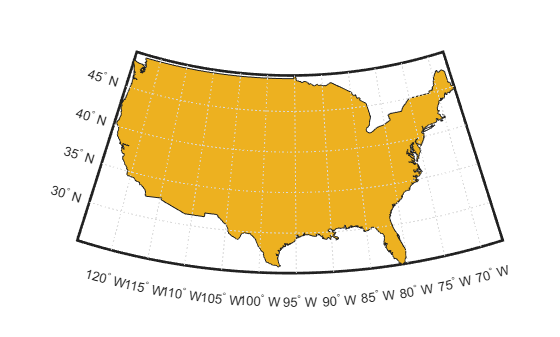# areaint

Surface area of polygon on sphere or ellipsoid

## Syntax

``a = areaint(lat,lon)``
``a = areaint(lat,lon,ellipsoid)``
``a = areaint(___,units)``

## Description

example

````a = areaint(lat,lon)` calculates the surface area of the polygon with geographic vertices `lat` and `lon`. This syntax references the coordinates to a unit sphere and returns `a` as the fraction of the sphere that the polygon covers.```

example

````a = areaint(lat,lon,ellipsoid)` specifies the reference ellipsoid for the vertices. This syntax returns the area in square units using the units of the semimajor axis of the reference ellipsoid.```
````a = areaint(___,units)` specifies the angle units for the vertices. ```

## Examples

collapse all

The accuracy of the `areaint` function depends on the distance between the specified vertices. Calculate more accurate approximations by specifying vertices that are closer together.

Specify vertices for a 30º lune, using a point every 30º of latitude. Approximate the surface area as the fraction of the unit sphere that the lune covers.

```lat1 = [-90:30:90 60:-30:-60]; lon1 = [zeros(1,7) 30*ones(1,5)]; a1 = areaint(lat1,lon1)```
```a1 = 0.0792 ```

Specify vertices for the same lune, this time using a point every 10º of latitude. Approximate the area of the lune using the new vertices.

```lat2 = [-90:10:90 80:-10:-80]; lon2 = [zeros(1,19) 30*ones(1,17)]; a2 = areaint(lat2,lon2)```
```a2 = 0.0829 ```

You can find the exact area of the lune by using the `areaquad` function. A 30º lune covers 1/12 the surface of the unit sphere.

`a = areaquad(90,0,-90,30)`
```a = 0.0833 ```

Load a MAT file containing coordinates for the conterminous United States, Long Island, and Martha's Vineyard into the workspace. Display the coordinates on a map using polygons.

```load("conus.mat","uslat","uslon") figure usamap([min(uslat) max(uslat)],[min(uslon) max(uslon)]) geoshow(uslat,uslon,"DisplayType","polygon")```Create a World Geodetic System of 1984 (WGS84) reference ellipsoid with a length unit of kilometers. Then, calculate the areas of the polygons in square kilometers by referencing the coordinates to the ellipsoid. In this case, the `areaint` function returns three areas. The largest area is for the polygon representing the conterminous United States. The other two areas are for the polygons representing Long Island and Martha's Vineyard, respectively.

```wgs84 = wgs84Ellipsoid("km"); a = areaint(uslat,uslon,wgs84)```
```a = 3×1 106 × 7.9326 0.0035 0.0004 ```

## Input Arguments

collapse all

Latitude coordinates of the polygon vertices, specified as a numeric vector.

• Define one polygon by specifying a vector, such as ```[39 45 19 39]```.

• Define multiple polygons by specifying a vector and indicating breaks between the polygons using `NaN` values, such as ```[37 46 31 20 37 NaN 45 49 35 32 45 NaN 35 40 42 35]```. The `NaN` values in `lat` must correspond to the `NaN` values in `lon`.

The size of `lat` must match the size of `lon`.

Data Types: `single` | `double`

Longitude coordinates of the polygon vertices, specified as a numeric vector.

• Define one polygon by specifying a vector, such as ```[39 45 19 39]```.

• Define multiple polygons by specifying a vector and indicating breaks between the polygons using `NaN` values, such as ```[37 46 31 20 37 NaN 45 49 35 32 45 NaN 35 40 42 35]```. The `NaN` values in `lon` must correspond to the `NaN` values in `lat`.

The size of `lon` must match the size of `lat`.

Data Types: `single` | `double`

Reference ellipsoid, specified as a `referenceSphere` object, a `referenceEllipsoid` object, an `oblateSpheroid` object, or a two-element vector of the form `[semimajor_axis eccentricity]`, where `semimajor_axis` is the length of the semimajor axis and `eccentricity` is the eccentricity. The values `semimajor_axis` and `eccentricity` must be of data type `double`.

The default value of `[1 0]` represents the unit sphere.

Angle unit for the vertices, specified as one of these options:

• `"degrees"` — Degrees

• `"radians"` — Radians

Data Types: `char` | `string`

## Output Arguments

collapse all

Surface area of the polygon, returned as a scalar or a vector.

• When `lat` and `lon` define one polygon, `a` is a scalar.

• When `lat` and `lon` define multiple polygons, `a` is a vector. Each element of the vector is the area of the corresponding polygon.

When you specify the `ellipsoid` argument, the area is in square units using the units of the semimajor axis of the reference ellipsoid. Otherwise, the area is the fraction of the unit sphere that the polygon covers.

Regardless of the polygon vertex order, the elements of `a` are positive.

Data Types: `double`

## Algorithms

• The `areaint` function measures areas enclosed by arbitrary polygons by using a line integral approach based on Green's Theorem. The accuracy is inversely proportional to the distance between the polygon vertices.

• When you specify `ellipsoid` as a nonspherical ellipsoid, the function converts the latitude data to the auxiliary authalic sphere.

## Version History

Introduced before R2006a